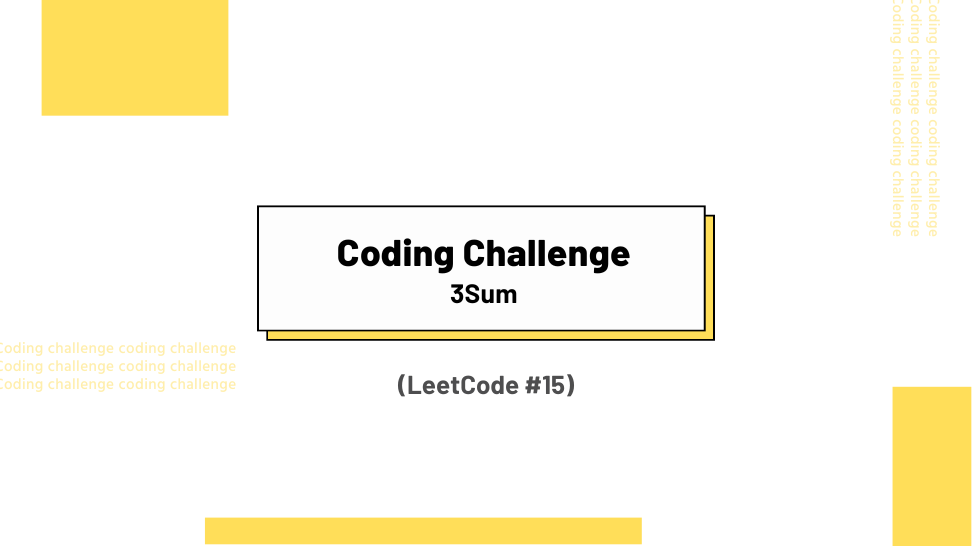## Challenge Statement

• Given an integer array nums, return all the triplets` [nums[i], nums[j], nums[k]] `such that `i != j, i != k, and j != k`, and `nums[i] + nums[j] + nums[k] == 0`.
• Notice that the solution set must not contain duplicate triplets.
• This challenge corresponds to LeetCode #15.

## Constraints

• `0 <= len(nums) <= 3000`
• `-105 <= nums[i] <= 105`

## Example 1:

Input: nums = `[-1 , 0, 1, 2, -1, -4]`

Output:` [[-1, -1, 2], [-1, 0, 1]]`

## Example 2:

Input:` nums = []`

Output: `[]`

## Example 3:

Input:` nums = `

Output: `[]`

## Solution

Below is my solution and some test cases. This solution has a quadratic time complexity O(n2) and a constant space complexity O(1), where n is the length of the input list.